omG8HI9LSKFW6lhCj8prTs0Z6lUhdOT9Jhi1Sf4m

# Three-Phase Electric Circuit: Balanced Wye-Delta Connection

After we learned about balanced wye-wye connection, we will learn about the most practical three-phase system. We usually use Y-connection three-phase sources with ∆-connected three-phase load.

As it implies, a balanced Y-∆ system has a balanced Y-connected source supplying a balanced ∆-connected load.

Make sure to read what is three-phase circuit first.

## Balanced Wye-Delta Connection

We can see the example of a balanced Y-∆ system in Figure.(1), where the source is Y-connection and the load is ∆-connection.Figure 1. Balanced Y-∆ connection
There is no neutral point from source to load for this connection. Assuming the positive sequence, the phase voltages are again(1)
The line voltages are(2)
Hence, it shows us that the voltage across the load impedances for this system is equal to the line voltages using this connection.

From these voltages, we can obtain the phase currents as(3)
These currents have the same magnitude but are out of phase with each other by 120◦.

Another way to get these phase currents is to apply KVL. For example, applying KVL around loop aABbna gives(4)
which is the same as Equation.(3). This is the more general way of finding the phase currents.

The line currents are obtained from the phase currents by applying KCL at nodes A, B, and C. Thus,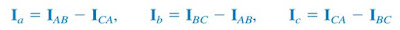(5)
Since ICAIAB∠−240◦,(6)
showing that the magnitude IL of the line current is √3 times the magnitude Ip of the phase current, or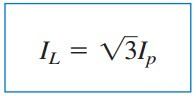(7)
where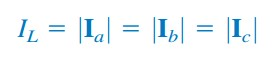(8)
and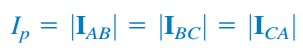(9)
Also, the line currents lag the corresponding phase currents by 30◦, assuming the positive sequence. Figure.(2) is a phasor diagram illustrating the relationship between the phase and line currents.Figure 2. Phasor diagram illustrating the relationship between phase and line currents
An alternative way of analyzing the Y-∆ circuit is to transform the ∆-connected load to an equivalent Y-connected load. Using the ∆-Y transformation formula,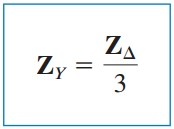(10)
After this transformation, we now have a Y-Y system as in the balanced wye-wye connection.

We can redraw the three-phase Y-∆ system in Figure.(1) to a single-phase equivalent circuit in Figure.(2). This allows us to calculate only the line currents. The phase currents are obtained using Equation.(7) and utilizing the fact that each of the phase currents leads the corresponding line current by 30◦.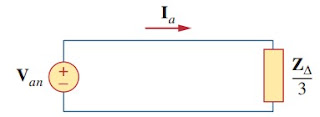Figure 3. A single-phase equivalent circuit of a balanced Y-∆ circuit

## Balanced Wye-Delta Connection Example

For better understanding let us review the example below:
1. A balanced abc-sequence Y-connected source with Van = 100∠10◦ V is connected to a ∆-connected balanced load (8 + j4) Ω per phase. We can get the phase and line currents.
Solution:
We can solve this problem in two ways.
Method 1
If the phase voltage Van = 100∠10◦, then the line voltage is
or
The phase currents are
The line currents are
Method 2
Alternatively, using single-phase analysis,
as above. Other line currents are obtained using the abc phase sequence.

Have you understood what is balanced wye-delta connection? Don't forget to share and subscribe! Happy learning!
Reference:  Fundamentals of electric circuits by Charles K. Alexander and Matthew N. O. Sadiku
Related Posts
SHARE